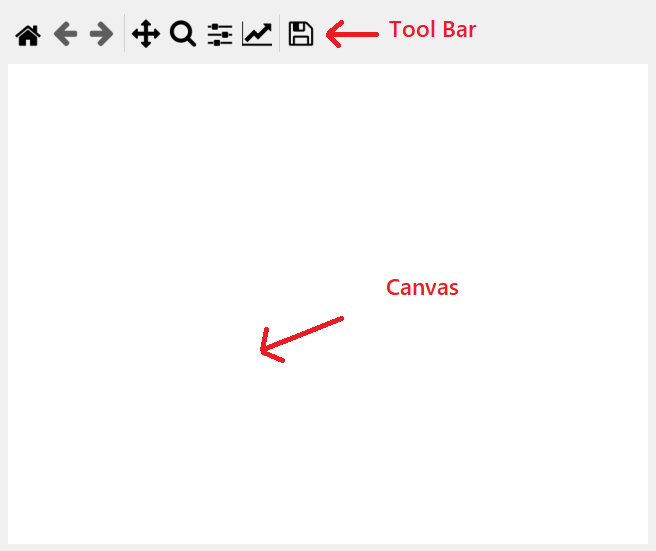# How to Embed Matplotlib Graph in PyQt5?

• Last Updated : 10 Jul, 2020

In this article, we will see how we can plot the graphs in the PyQt5 window using matplotlib.

Matplotlib is an amazing visualization library in Python for 2D plots of arrays. Matplotlib is a multi-platform data visualization library built on NumPy arrays and designed to work with the broader SciPy stack. It was introduced by John Hunter in the year 2002.

Attention geek! Strengthen your foundations with the Python Programming Foundation Course and learn the basics.

To begin with, your interview preparations Enhance your Data Structures concepts with the Python DS Course. And to begin with your Machine Learning Journey, join the Machine Learning - Basic Level Course

PyQt5 is cross-platform GUI toolkit, a set of Python bindings for Qt v5. One can develop an interactive desktop application with so much ease because of the tools and simplicity provided by this library. A GUI application consists of Front-end and Back-end.

## Getting Started

In order to plot graphs using Matplotlib in PyQt5 we need `FigureCanvasQTAgg and NavigationToolbar2QT` these are similar to the PyQt5 widgets these are embedding.

• NavigationToolbar2QT : It will provide the tool bar for the graph, It can be imported with the help of command given below

• FigureCanvasQTAgg : It will provide the canvas for the graph, It can be imported with the help of command given below

from matplotlib.backends.backend_qt5agg import FigureCanvasQTAgg as FigureCanvas

Below is how `FigureCanvasQTAgg and NavigationToolbar2QT` looks like –Below is the implementation

 `# importing various libraries``import` `sys``from` `PyQt5.QtWidgets ``import` `QDialog, QApplication, QPushButton, QVBoxLayout``from` `matplotlib.backends.backend_qt5agg ``import` `FigureCanvasQTAgg as FigureCanvas``from` `matplotlib.backends.backend_qt5agg ``import` `NavigationToolbar2QT as NavigationToolbar``import` `matplotlib.pyplot as plt``import` `random``  ` `# main window``# which inherits QDialog``class` `Window(QDialog):``      ` `    ``# constructor``    ``def` `__init__(``self``, parent``=``None``):``        ``super``(Window, ``self``).__init__(parent)``  ` `        ``# a figure instance to plot on``        ``self``.figure ``=` `plt.figure()``  ` `        ``# this is the Canvas Widget that ``        ``# displays the 'figure'it takes the``        ``# 'figure' instance as a parameter to __init__``        ``self``.canvas ``=` `FigureCanvas(``self``.figure)``  ` `        ``# this is the Navigation widget``        ``# it takes the Canvas widget and a parent``        ``self``.toolbar ``=` `NavigationToolbar(``self``.canvas, ``self``)``  ` `        ``# Just some button connected to 'plot' method``        ``self``.button ``=` `QPushButton(``'Plot'``)``          ` `        ``# adding action to the button``        ``self``.button.clicked.connect(``self``.plot)``  ` `        ``# creating a Vertical Box layout``        ``layout ``=` `QVBoxLayout()``          ` `        ``# adding tool bar to the layout``        ``layout.addWidget(``self``.toolbar)``          ` `        ``# adding canvas to the layout``        ``layout.addWidget(``self``.canvas)``          ` `        ``# adding push button to the layout``        ``layout.addWidget(``self``.button)``          ` `        ``# setting layout to the main window``        ``self``.setLayout(layout)``  ` `    ``# action called by thte push button``    ``def` `plot(``self``):``          ` `        ``# random data``        ``data ``=` `[random.random() ``for` `i ``in` `range``(``10``)]``  ` `        ``# clearing old figure``        ``self``.figure.clear()``  ` `        ``# create an axis``        ``ax ``=` `self``.figure.add_subplot(``111``)``  ` `        ``# plot data``        ``ax.plot(data, ``'*-'``)``  ` `        ``# refresh canvas``        ``self``.canvas.draw()``  ` `# driver code``if` `__name__ ``=``=` `'__main__'``:``      ` `    ``# creating apyqt5 application``    ``app ``=` `QApplication(sys.argv)``  ` `    ``# creating a window object``    ``main ``=` `Window()``      ` `    ``# showing the window``    ``main.show()``  ` `    ``# loop``    ``sys.exit(app.exec_())`

Output :

My Personal Notes arrow_drop_up